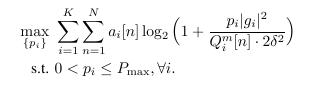# How can I solve this kind of large scale problem?

I am stuck in this problembecause it is solved quite slow. My code is as following:
cvx_begin
variables pm1 pm2 pm3 pm4 pm5 pm6
maximize sum(am1.*log(1+pm1.*C1’))+sum(am2.*log(1+pm2.*C2’))+ sum(am3.*log(1+pm3.*C3’))+ sum(am4.*log(1+pm4.*C4’))+ sum(am5.*log(1+pm5.*C5’))+ sum(am6.*log(1+pm6.*C6’))
subject to
0<pm1<=P_max;
0<pm2<=P_max;
0<pm3<=P_max;
0<pm4<=P_max;
0<pm5<=P_max;
0<pm6<=P_max;
cvx_end
where C_i=|g_i|^2/{Q_i^m[n]*2*\delta^2}.

I takes a quite long time to perform the problem, Somtimes my computer even crashed due to the running so that I had to terminate it.

I think the problem results from the functions ‘log’ which requires successive approximation. So could somebody suggest an equivalent formulation that CVX can process more efficiently?

Try using CVXQUAD per my April post in CVX reports unbounded status but with viable solution . You will have to rewrite log in terms of explicit exponential cone cone construct, as mentioned there (also see mvg’s answer at Solve optimization problems of exp function for reformulation).

Thanks a lot!

But I don’t think I completely understand your suggestion. Could you please give a further help?

For each term, log(x), replace it with an additional CVX variable z and add the constraint
{z,1,x} == exponential(1)
which models exp(z) <= x, i.e., models z <= log(x)

So you are going to have to add a lot of variables and constraints. However, I think you can vectorize the exponential cone constraints as follows:

variables x(n) z(n)
{z,1,x} == exponential(n)


rather than using n separate constraints of the form
{z(i),1,x(i)} == exponential(1)

Instead of the exponential cone construct, I believe you can use a vectroized rel_entr constraint

variables x(n) z(n)
z + rel_entr(1,x) <= 0


and this will be sufficient to invoke CVXQUAD’s Pade approximation in lieu of CVX’s successive approximation method.

I suggest you start with a simplified version of your problem to make sure everything is working correctly. Then go to the full version of your problem.

Edit: Things can be done more simply than I showed. Each instance of log(x) can be replaced by -rel_entr(1,x) without requiring any additional variables or constraints. This should work for scalar, vector, or even matrix argument o f log. That will be sufficient to invoke CVXQUAD’s Pade approximant in lieu of CVX’s successive approximation method. This still requires CVX QUAD to be installed, including its exponential.m replacement.

1 Like